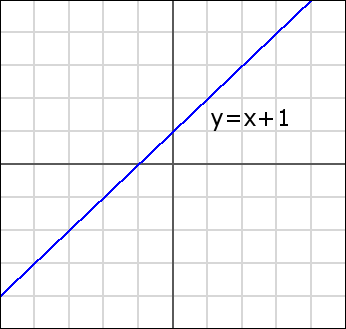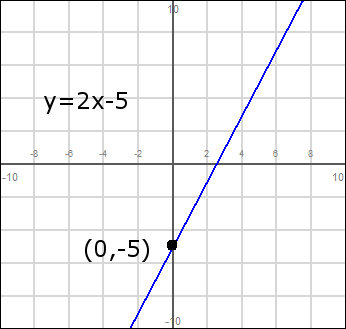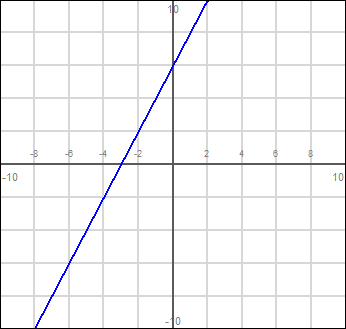# What is slope-intercept form?

It's a standard method for writing the equation of a line. It has two variables, x and y, and an unlimited number of "solutions" (the line is infinitely long).

### What does it look like?

A line written in slope-intercept form takes this form:

$$\huge y=mx+b$$

Sometimes you'll even hear people refer to slope-intercept as "y=mx+b form" because it's so well known. Let's examine what each term means. Remember that you are plotting this graph on an x-y plane, so the variables x and y represent geometric points on the graph. The constant 'm' is the slope of the graph -- notice how it multiplies your x term. That's because the slope of a graph determines how much 'y' changes as a function of 'x'. The final term, b, is the y-intercept. That's the point at which the line crosses the y-axis, where x=0. If you plug in 0 for x, you'll see that all you have is y=b.

### An example:

Q: Plot the line described by this equation: $$y=x+1$$

A: What is the slope of this line? It's the 'm' term in y=mx+b. In this example, m=1. Therefore the slope of the line is 1, or said another way, the line goes "up" one unit every time it goes "over" one unit.

What is the y-intercept of the line? That's the 'b' term, so it's also 1. That means that when x is 0, y=1. Go ahead and plug x=0 into that equation to verify for yourself.In that example, notice how the line crosses the y-axis (the vertical axis) at y=1? That's the "intercept" part of slope-intercept form.

### Let's see something more complicated.

The next example will have some more complicated numbers. However, it's still as simple as reading them from the equation and working through it step-by-step.

Q: Plot the line $$y = 2x - 5$$

A: Ok, so what is the slope? That the 'm', and multiplies the x. In this case it's 2. What's the y-intercept? Notice that it's being subtracted, so the y-intercept is actually -5.

Ok, so how do you actually plot that??

You can start from the y-intercept. We know the line crosses the y-axis at $$y=-5$$, so you can plot the point $$(0,-5)$$.

Then, since the slope is 2, you need to go up two units for every 1 you go over:Ok, so do you understand the basics now? Just find the y-intercept, and then draw a line with the given slope.

But, sometimes the equation won't be in an easy-to-read $$y=mx+b$$ format. Sometimes it will look more complicated. All you have to do is rewrite the equation into that form, like this:

### Re-writing into point-slope form:

Q: Plot the line $$y=2*(x+3)$$.

A: Rewrite into $$y=mx+b$$ format. Multiply the 2 over the parenthesis:

$$y=2*(x+3)$$ $$y=2x+6$$

Now we're in the right format, and we can easily plot itSometimes you will see the x and y on the same side of the equation, or a term that multiplies y, like this nasty example:

$$4y+x=12$$

Like before, just use your basic algebra knowledge to rewrite into $$y=mx+b$$ format:

$$4y+x = 12$$ $$4y = -x+12$$ $$y = -.25x+3$$

Getting the basic hang of it? Check out a similar method of graphing lines, the point-slope format. Or read more lessons on slope intercept from other websites.